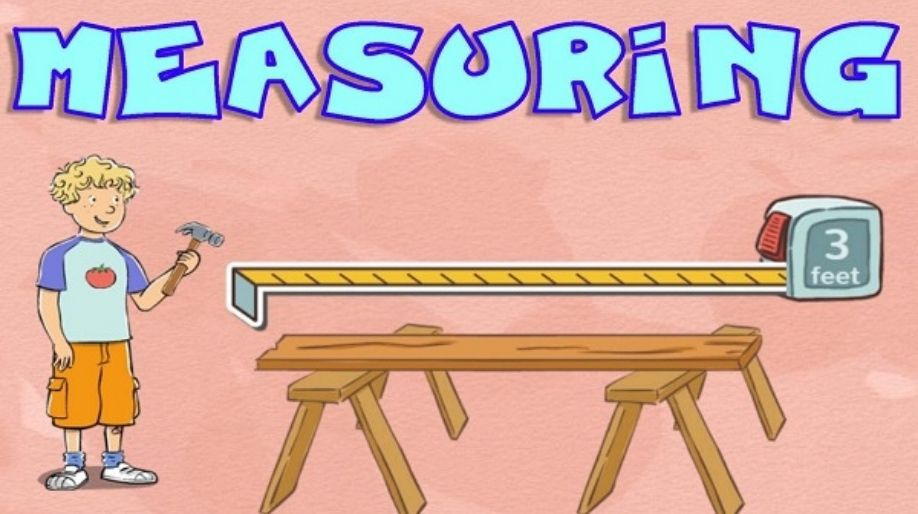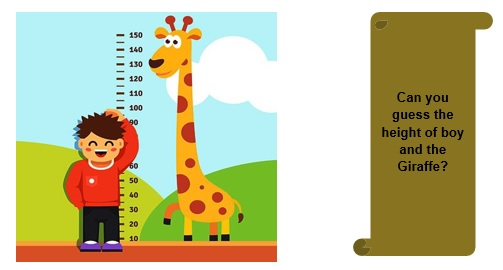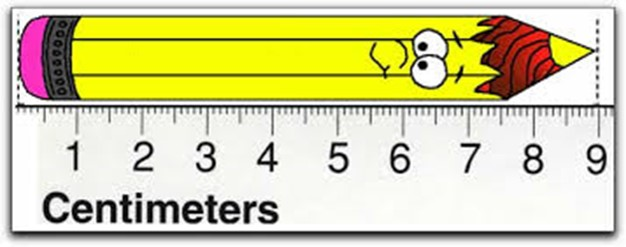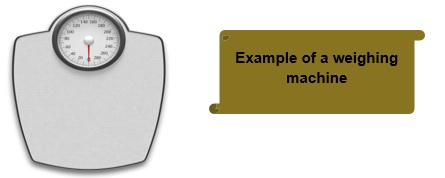Register for Maths, Science, English, Reasoning Olympiad Exams Register here | Book Free Demo for Live Olympiad Classes here | Check Olympiad Exam Dates here | Buy Practice Papers for IMO, IOM, HEO, IOEL etc here | Login here to participate in all India free mock test on every Saturday

# Length and WeightWhat is length and weight? We all come up with a question “What is your height"? And we all answer it accordingly. For example my height is 185 cm. But again the question arises what is height actually? Let’s find out the answer to all these questions.Length is actually the measurement of how long is something.

• As mentioned above my height is 185 cm or I can say that I am 185 cm long.
• Here my measurement of height is my length.

Now all of you will ask this question to each other and we all get to know each other’s height. Isn’t it interesting?

So let’s explore more about the topic.

• There are instruments to measure the length of any body like rulers (scales), tapes etc.
• The length is always measured in mm (millimeter), cm (centimeters), m (meters), km (kilometers) etc. These are the units of length.
• The standard unit of length is Meter.
• For shorter length like of a pencil we use the unit cm, for a bigger length like of a person we use the unit m, and for a larger length like of a road we use the unit km.

Conversions

The changing of unit into the other using some mathematical formulas are known as conversions.

• The smallest unit to measure a distance is millimeter (mm).

1 mm = 0.1 cm = 0.001 m

Example 1:- The length of tip of your pencil is 5mm or 0.5 cm.

• The next unit to measure length is centimeter (cm).

1 cm = 0.01 m and 1 cm = 10 mm

Example 2:- The length of your pencil is 20 cm.

Therefore in mm it would be 20 x 10 = 200mm.

• The next unit to measure length is meter (m).

1 m = 100 cm = 1000mm.

Example 3:- The length of your classroom is 7 m.

Therefore it will be 700 cm and 7000 mm.

• The largest unit to measure length is km.

1 km = 1000 m = 100000 cm = 1000000 mm.

Example 4:- The length of Narmada River is 1312 km.

It equals to 1312 x 1000 m = 1312000 m.

Let us now find where and how we are going use these concepts which we have learned till now.

Example 5:- Convert 3 m into cm.

Solution:- As we know that 1 m = 100 cm.

Therefore 3 m = (3 x 100) cm = 300 cm.

Example 6:- Convert 5.32 m into cm.

Solution:- As we know that 1 m = 100 cm.

Therefore 5.32 m = (5.32 x 100) cm = 532 cm.

Example 7:- A shopkeeper has 128 m of wire. He sold 23 m wire to a customer. How much length of wire is he left with?

Solution:- Length of wire before selling = 128 m

Length of wire sold = 23 m

Length of wire remaining is = (128-23) m = 105 m.

Example 8:- Identify the length of pencil with the help of scale.Here the length of pencil can be identified as 9 cm.

Practice questions

Question 1:- Convert the following as directed.

1. 5 cm into mm.
2. 32 cm into mm.
3. 123 m into mm.
4. 52 km into m.

Question 2:- Ajay has ropes of 3 colors Red, Blue and Black. The Red rope is 23 m long; the Black rope is 12m long. Ajay has to measure a length of 69 m with these ropes. How much length of Blue rope is required?

Question 3:- State True or False:

1. The smallest unit of length is cm.
2. 1 cm = 100 m
3. 1 km = 1000 cm.
4. 3 m = 330mm

Weight

We all again come up with a question “What is your weight". And we answer it accordingly. I will answer it by saying “My weight is 71 Kg." But still the question arises what actually weight is? Let’s just find out the answers.

• Weight is actually the measurement of how heavy is something.
• As mentioned above I am 71 kg heavy or my weight is 71 kg.

Let’s do a activity and ask each other how heavy they are.

And let’s explore more about the topic weight.• The instruments used to measure the weight are Weighing machines, balance scales etc.
• The units of weight are g (Gram) and kg (Kilogram).
• The standard unit of weight is Kilogram.

For lighter objects like for weighing of a mobile phone we use the unit Gram and for weighing heavier objects like for weighing of an Elephant we use the unit Kilogram.

Conversions

The changing of units into one another using some mathematical formulae are known as conversions.

• 1 g = 0.001 Kg or 1/1000 gm.

Example 1:- The mass of an object is 250 gm. What will it be in Kilograms?

Solution:- As we know that 1 g = 0.001 kg

Therefore 250 g = (250 x 0.001) kg = 0.250 kg.

• 1 Kg = 1000 g.

Example 2:- The weight of an object is 12 kg. Convert it into grams.

Solution:- As we know that 1 kg = 1000 g.

Therefore 12 kg = (12 x 1000) g = 12000 g.

Example 3:- Ram is going to a market to purchase sugar and Flour. Ram has a carry bag which can sustain a max weight of 14 kg and 300 g. If Ram has to buy 6 kg and 200 g of sugar then how much flour can he purchase so that he can take both of them inside the carry bag back to home?

Solution:- Weight of sugar = 6 kg and 200 g.

Maximum weight that can be kept inside the carry bag

= 14 kg and 300 g.

Therefore without damaging the carry bag the weight of flour purchased must be =

(Max weight) – (Weight of Sugar)

= 14 kg – 6 kg and 300 g – 200 g

= 8 kg and 100 g.

Hence Ram can purchase maximum of 8 kg and 100 g of flour.

Practice Questions

Question 1:- Convert 23.23 kg into g .

Question 2:- Convert 6300 g into Kg.

Question 3:- State True or False:

1. 1 kg = 1000 g
2. 200 g =0.2 kg
3. 2 kg = 42000 g

Question 4:- The weight of Ajay is 30 kg and the weight of Raman is 28 kg. Who is heavier and by how much?

Recap

• Length and Weight are the measurement quantities.
• The standard unit of length is meter and weight is Kg.
• 1 km = 1000 m and 1 m = 100 cm.
• 1 kg = 1000 g.
• For shorter distance we use the unit m or cm and for longer distance we use the unit km.

For lighter objects we use the unit g and for the heavier objects we use the unit Kg.

## Quiz for Length and Weight

 Q.1 SI unit of length is _________. a) km b) cm c) m d) mm
 Q.2 SI unit of weight is ________. a) Kg b) Gm c) Mg d) Newton
 Q.3 CGS unit of length is _______. a) km b) cm c) m d) mm
 Q.4 CGS unit of weight is ______. a) kg b) mg c) dg d) g
 Q.5 1 km = __________ m. a) 100 b) 10 c) 1000 d) 10000
 Q.6 1 m = __________ cm. a) 100 b) 10 c) 1000 d) 10000
 Q.7 1 kg = _______ g. a) 100 b) 0.001 c) 10000 d) 1000
 Q.8 1 g = _______ mg. a) 100 b) 1000 c) 10 d) 0.001
 Q.9 The most widely used unit for the measurement of distance is _____. a) m b) km c) cm d) mm
 Q.10 1 foot = ____ inches. a) 100 b) 10 c) 12 d) 24